# Fcv hum mach_geman

30 de Dec de 2011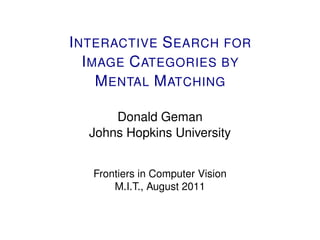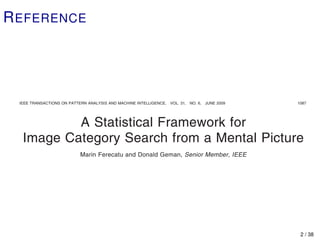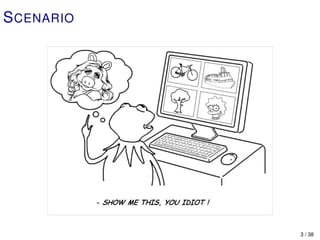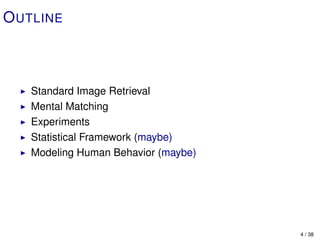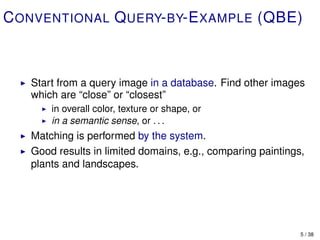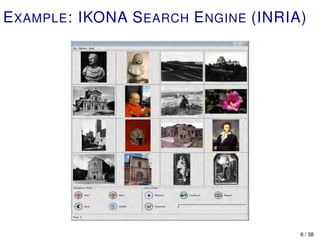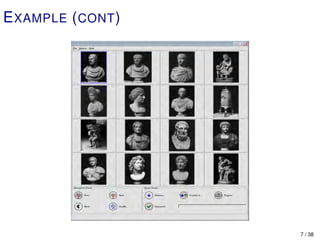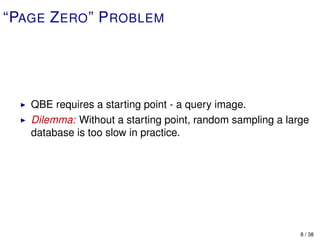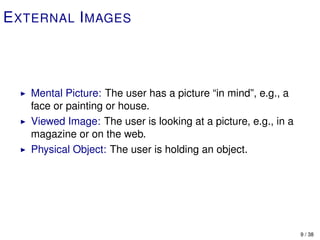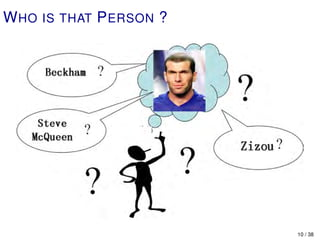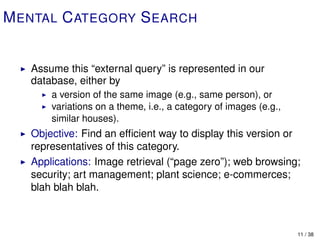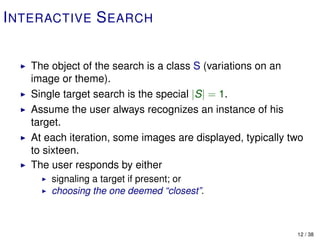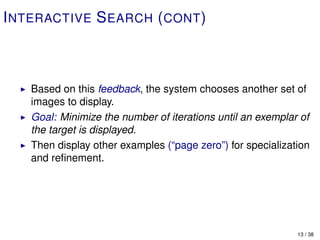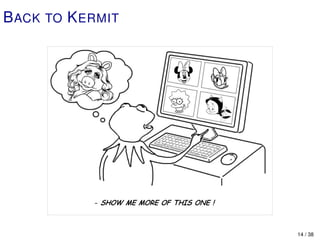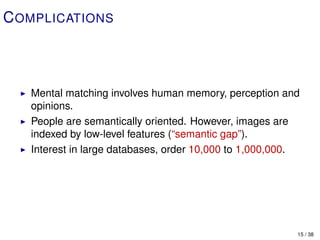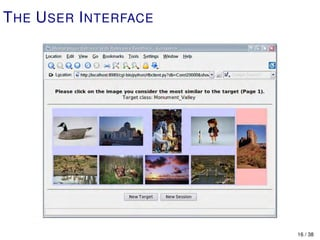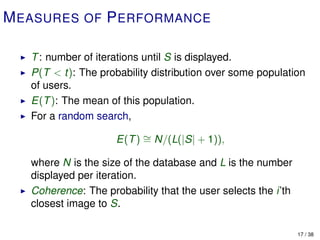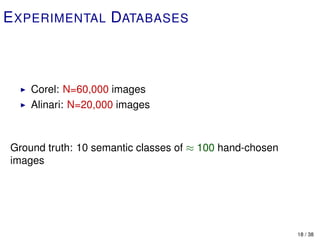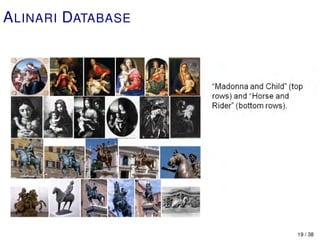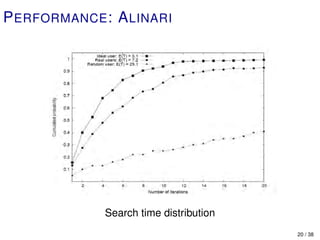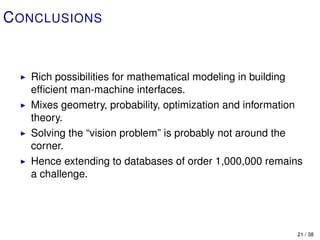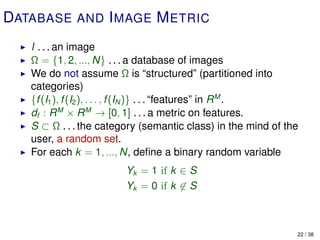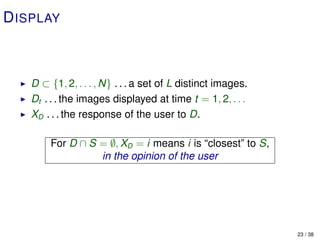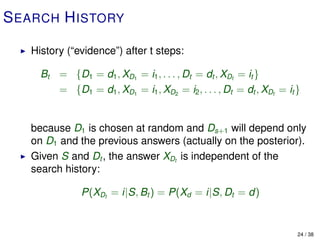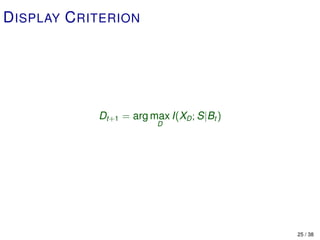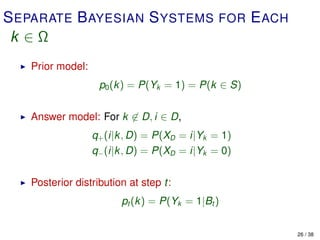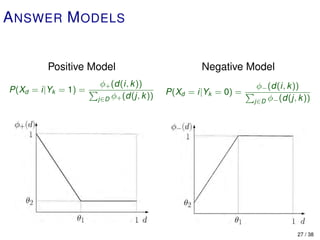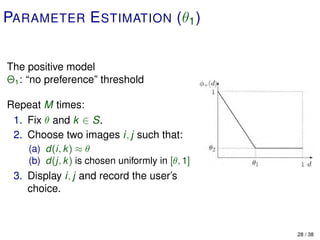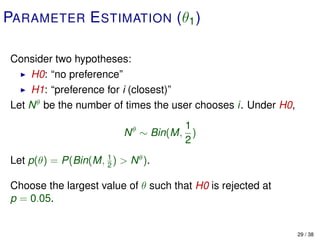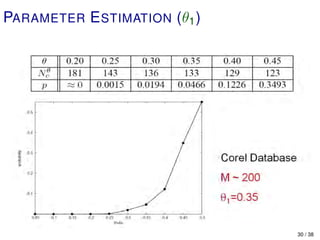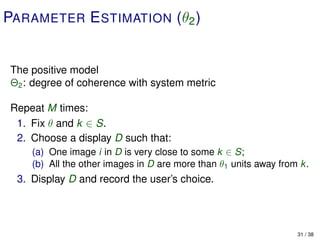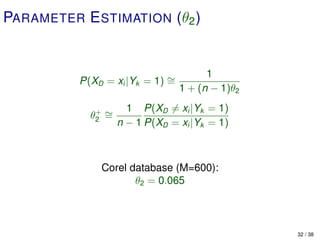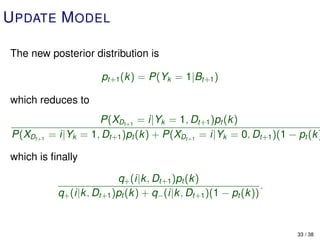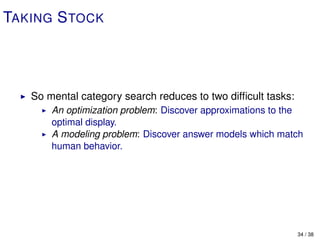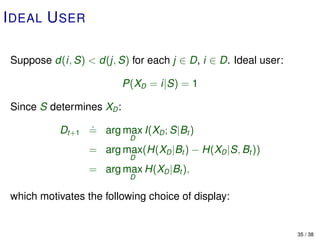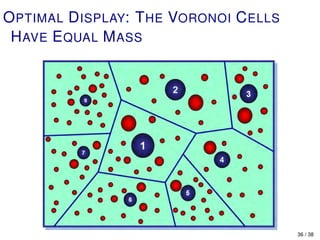1 de 36

### Fcv hum mach_geman

• 1. I NTERACTIVE S EARCH FOR I MAGE C ATEGORIES BY M ENTAL M ATCHING Donald Geman Johns Hopkins University Frontiers in Computer Vision M.I.T., August 2011
• 2. R EFERENCE IEEE TRANSACTIONS ON PATTERN ANALYSIS AND MACHINE INTELLIGENCE, VOL. 31, NO. 6, JUNE 2009 1087 A Statistical Framework for Image Category Search from a Mental Picture Marin Ferecatu and Donald Geman, Senior Member, IEEE Abstract—Starting from a member of an image database designated the “query image,” traditional image retrieval techniques, for example, search by visual similarity, allow one to locate additional instances of a target category residing in the database. However, in many cases, the query image or, more generally, the target category, resides only in the mind of the user as a set of subjective visual patterns, psychological impressions, or “mental pictures.” Consequently, since image databases available today are often unstructured and lack reliable semantic annotations, it is often not obvious how to initiate a search session; this is the “page zero problem.” We propose a new statistical framework based on relevance feedback to locate an instance of a semantic category in an unstructured image database with no semantic annotation. A search session is initiated from a random sample of images. At each retrieval round, the user is asked to select one image from among a set of displayed images—the one that is closest in his opinion to the target class. The matching is then “mental.” Performance is measured by the number of iterations necessary to display an image which satisfies the user, at which point standard techniques can be employed to display other instances. Our core contribution is a Bayesian formulation which scales to large databases. The two key components are a response model which accounts for the user’s subjective perception of similarity and a display algorithm which seeks to maximize the flow of information. Experiments with real users and two databases of 2 / 38
• 3. S CENARIO 3 / 38
• 4. O UTLINE Standard Image Retrieval Mental Matching Experiments Statistical Framework (maybe) Modeling Human Behavior (maybe) 4 / 38
• 5. C ONVENTIONAL Q UERY- BY-E XAMPLE (QBE) Start from a query image in a database. Find other images which are “close” or “closest” in overall color, texture or shape, or in a semantic sense, or . . . Matching is performed by the system. Good results in limited domains, e.g., comparing paintings, plants and landscapes. 5 / 38
• 6. E XAMPLE : IKONA S EARCH E NGINE (INRIA) 6 / 38
• 7. E XAMPLE ( CONT ) 7 / 38
• 8. “PAGE Z ERO ” P ROBLEM QBE requires a starting point - a query image. Dilemma: Without a starting point, random sampling a large database is too slow in practice. 8 / 38
• 9. E XTERNAL I MAGES Mental Picture: The user has a picture “in mind”, e.g., a face or painting or house. Viewed Image: The user is looking at a picture, e.g., in a magazine or on the web. Physical Object: The user is holding an object. 9 / 38
• 10. W HO IS THAT P ERSON ? 10 / 38
• 11. M ENTAL C ATEGORY S EARCH Assume this “external query” is represented in our database, either by a version of the same image (e.g., same person), or variations on a theme, i.e., a category of images (e.g., similar houses). Objective: Find an efﬁcient way to display this version or representatives of this category. Applications: Image retrieval (“page zero”); web browsing; security; art management; plant science; e-commerces; blah blah blah. 11 / 38
• 12. I NTERACTIVE S EARCH The object of the search is a class S (variations on an image or theme). Single target search is the special |S| = 1. Assume the user always recognizes an instance of his target. At each iteration, some images are displayed, typically two to sixteen. The user responds by either signaling a target if present; or choosing the one deemed “closest”. 12 / 38
• 13. I NTERACTIVE S EARCH ( CONT ) Based on this feedback, the system chooses another set of images to display. Goal: Minimize the number of iterations until an exemplar of the target is displayed. Then display other examples (“page zero”) for specialization and reﬁnement. 13 / 38
• 14. B ACK TO K ERMIT 14 / 38
• 15. C OMPLICATIONS Mental matching involves human memory, perception and opinions. People are semantically oriented. However, images are indexed by low-level features (“semantic gap”). Interest in large databases, order 10,000 to 1,000,000. 15 / 38
• 16. T HE U SER I NTERFACE 16 / 38
• 17. M EASURES OF P ERFORMANCE T : number of iterations until S is displayed. P(T < t): The probability distribution over some population of users. E(T ): The mean of this population. For a random search, E(T ) ∼ N/(L(|S| + 1)), = where N is the size of the database and L is the number displayed per iteration. Coherence: The probability that the user selects the i’th closest image to S. 17 / 38
• 18. E XPERIMENTAL DATABASES Corel: N=60,000 images Alinari: N=20,000 images Ground truth: 10 semantic classes of ≈ 100 hand-chosen images 18 / 38
• 19. A LINARI DATABASE 19 / 38
• 20. P ERFORMANCE : A LINARI Search time distribution 20 / 38
• 21. C ONCLUSIONS Rich possibilities for mathematical modeling in building efﬁcient man-machine interfaces. Mixes geometry, probability, optimization and information theory. Solving the “vision problem” is probably not around the corner. Hence extending to databases of order 1,000,000 remains a challenge. 21 / 38
• 22. DATABASE AND I MAGE M ETRIC I . . . an image Ω = {1, 2, ..., N} . . . a database of images We do not assume Ω is “structured” (partitioned into categories) {f (I1 ), f (I2 ), . . . , f (IN )} . . . “features” in R M . df : R M × R M → [0, 1] . . . a metric on features. S ⊂ Ω . . . the category (semantic class) in the mind of the user, a random set. For each k = 1, ..., N, deﬁne a binary random variable Yk = 1 if k ∈ S Yk = 0 if k ∈ S 22 / 38
• 23. D ISPLAY D ⊂ {1, 2, . . . , N} . . . a set of L distinct images. Dt . . . the images displayed at time t = 1, 2, . . . XD . . . the response of the user to D. For D ∩ S = ∅, XD = i means i is “closest” to S, in the opinion of the user 23 / 38
• 24. S EARCH H ISTORY History (“evidence”) after t steps: Bt = {D1 = d1 , XD1 = i1 , . . . , Dt = dt , XDt = it } = {D1 = d1 , XD1 = i1 , XD2 = i2 , . . . , Dt = dt , XDt = it } because D1 is chosen at random and Ds+1 will depend only on D1 and the previous answers (actually on the posterior). Given S and Dt , the answer XDt is independent of the search history: P(XDt = i|S, Bt ) = P(Xd = i|S, Dt = d) 24 / 38
• 25. D ISPLAY C RITERION Dt+1 = arg max I(XD ; S|Bt ) D 25 / 38
• 26. S EPARATE B AYESIAN S YSTEMS FOR E ACH k ∈Ω Prior model: p0 (k) = P(Yk = 1) = P(k ∈ S) Answer model: For k ∈ D, i ∈ D, q+ (i|k, D) = P(XD = i|Yk = 1) q− (i|k, D) = P(XD = i|Yk = 0) Posterior distribution at step t: pt (k) = P(Yk = 1|Bt ) 26 / 38
• 27. A NSWER M ODELS Positive Model Negative Model φ+ (d(i, k )) φ− (d(i, k )) P(Xd = i|Yk = 1) = P(Xd = i|Yk = 0) = j∈D φ+ (d(j, k )) j∈D φ− (d(j, k )) 27 / 38
• 28. PARAMETER E STIMATION (θ1 ) The positive model Θ1 : “no preference” threshold Repeat M times: 1. Fix θ and k ∈ S. 2. Choose two images i, j such that: (a) d(i, k ) ≈ θ (b) d(j, k ) is chosen uniformly in [θ, 1] 3. Display i, j and record the user’s choice. 28 / 38
• 29. PARAMETER E STIMATION (θ1 ) Consider two hypotheses: H0: “no preference” H1: “preference for i (closest)” Let N θ be the number of times the user chooses i. Under H0, 1 N θ ∼ Bin(M, ) 2 Let p(θ) = P(Bin(M, 1 ) > N θ ). 2 Choose the largest value of θ such that H0 is rejected at p = 0.05. 29 / 38
• 30. PARAMETER E STIMATION (θ1 ) 30 / 38
• 31. PARAMETER E STIMATION (θ2 ) The positive model Θ2 : degree of coherence with system metric Repeat M times: 1. Fix θ and k ∈ S. 2. Choose a display D such that: (a) One image i in D is very close to some k ∈ S; (b) All the other images in D are more than θ1 units away from k. 3. Display D and record the user’s choice. 31 / 38
• 32. PARAMETER E STIMATION (θ2 ) 1 P(XD = xi |Yk = 1) ∼ = 1 + (n − 1)θ2 1 P(XD = xi |Yk = 1) θ2 ∼ + = n − 1 P(XD = xi |Yk = 1) Corel database (M=600): θ2 = 0.065 32 / 38
• 33. U PDATE M ODEL The new posterior distribution is pt+1 (k) = P(Yk = 1|Bt+1 ) which reduces to P(XDt+1 = i|Yk = 1, Dt+1 )pt (k) P(XDt+1 = i|Yk = 1, Dt+1 )pt (k) + P(XDt+1 = i|Yk = 0, Dt+1 )(1 − pt (k ) which is ﬁnally q+ (i|k, Dt+1 )pt (k ) . q+ (i|k, Dt+1 )pt (k) + q− (i|k, Dt+1 )(1 − pt (k)) 33 / 38
• 34. TAKING S TOCK So mental category search reduces to two difﬁcult tasks: An optimization problem: Discover approximations to the optimal display. A modeling problem: Discover answer models which match human behavior. 34 / 38
• 35. I DEAL U SER Suppose d(i, S) < d(j, S) for each j ∈ D, i ∈ D. Ideal user: P(XD = i|S) = 1 Since S determines XD : . Dt+1 = arg max I(XD ; S|Bt ) D = arg max(H(XD |Bt ) − H(XD |S, Bt )) D = arg max H(XD |Bt ), D which motivates the following choice of display: 35 / 38
• 36. O PTIMAL D ISPLAY: T HE VORONOI C ELLS H AVE E QUAL M ASS 36 / 38